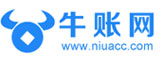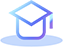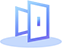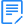# excel中引用函数计算公式，赶紧收藏！1、查询重复内容公式

=IF(COUNTIF(A:A,A2)>1,"重复","")

2、查询重复身份证号码公式

=IF(COUNTIF(A:A,A2&"*")>1,"重复","")

3、依照身份证号计算出生年月公式

=TEXT(MID(A2,7,8),"0!/00!/00")

4、使用出生年月来算年龄公式

=DATEDIF(A2,TODAY(),"y")

5、按照身份证号码提取性别公式

=IF(MOD(MID(A2,15,3),2),"男","女")

6、依照身份证号码计算退休时间

=EDATE(TEXT(MID(A2,7,8),"0!/00!/00"),MOD(MID(A2,15,3),2)*120+600)

7、计算某个日期是第几季度

=LEN(2^MONTH(A2))&"季度"

8、条件求和

=SUMIF(B:B,"一部",C:C)

9、多条件求和

=SUMIFS(C:C,B:B,"一部",C:C,">2700")

10、计算合同到期公式

=EDATE(A2,3)

## 限时资料免费

•重点汇总

•历年真题

•章节练习

•备考攻略

•高分经验

•预测密卷

## 推荐课程